#Function Repository Resource:

# SCombinatorHaltsQ

Test whether evolution of an S combinator expression will halt

Contributed by: Wolfram Research
 ResourceFunction["SCombinatorHaltsQ"][cmb] check whether the S combinator expression cmb will terminate.

## Details and Options

The combinator expression cmb should be given in nested-bracket form and contain only the S combinator.
ResourceFunction["SCombinatorHaltsQ"] accepts one option:
 "SGlyph" symbol used to represent the S combinator in cmb

## Examples

### Basic Examples (2)

Demonstrate that an S combinator expression halts:

 In:=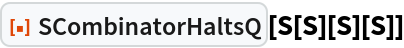Out=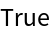This corresponds to the fact that its evolution reaches a fixed point after one step:

 In:=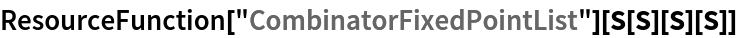Out=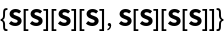Define an S combinator expression with a leaf count that increases with each step:

 In:=Out=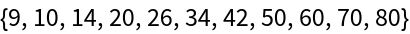Thus its evolution does not terminate:

 In:=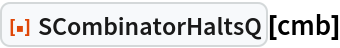Out=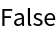### Options (2)

Define symbols other than the default CombinatorS glyph to represent the S combinator:

 In:=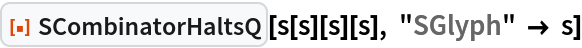Out=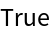In:=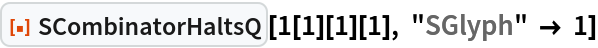Out=SCombinatorHaltsQ throws a failure when the input does not use the specified "SGlyph":

 In:=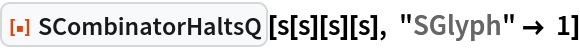Out=The input expression should exclusively use the S combinator:

 In:=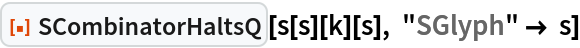Out=